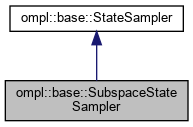ompl::base::SubspaceStateSampler Class Reference

Construct a sampler that samples only within a subspace of the space. More...

#include <ompl/base/StateSampler.h>

Inheritance diagram for ompl::base::SubspaceStateSampler:[legend]

## Public Member Functions

SubspaceStateSampler (const StateSpace *space, const StateSpace *subspace, double weight)
Construct a sampler for space but only sample components common to subspace. Use weight as a multiplicative factor for distance and stdDev in the sampleUniformNear() and sampleGaussian() functions.

void sampleUniform (State *state) override
Sample a state.

void sampleUniformNear (State *state, const State *near, double distance) override
Sample a state near another, within a neighborhood controlled by a distance parameter. More...

void sampleGaussian (State *state, const State *mean, double stdDev) override
Sample a state using a Gaussian distribution with given mean and standard deviation (stdDev). More...Public Member Functions inherited from ompl::base::StateSampler
StateSampler (const StateSampler &)=delete

StateSampleroperator= (const StateSampler &)=delete

StateSampler (const StateSpace *space)
Constructor.

## Protected Attributes

const StateSpacesubspace_
The subspace to sample.

StateSamplerPtr subspaceSampler_
The sampler for the subspace.

double weight_
The weigth factor to multiply distance and stdDev when sampling in the vicinity of a state.

std::vector< std::string > subspaces_
The names of common subspaces between space_ and subspace_; these are the ones copied after sampling a state.Protected Attributes inherited from ompl::base::StateSampler
const StateSpacespace_
The state space this sampler samples.

RNG rng_
An instance of a random number generator.

## Detailed Description

Construct a sampler that samples only within a subspace of the space.

Definition at line 153 of file StateSampler.h.

## ◆ sampleGaussian()

 void ompl::base::SubspaceStateSampler::sampleGaussian ( State * state, const State * mean, double stdDev )
overridevirtual

Sample a state using a Gaussian distribution with given mean and standard deviation (stdDev).

As with sampleUniform, the implementation of sampleGaussian is specific to the derived class and few assumptions can be made about the distance between state and mean.

Implements ompl::base::StateSampler.

Definition at line 104 of file StateSampler.cpp.

## ◆ sampleUniformNear()

 void ompl::base::SubspaceStateSampler::sampleUniformNear ( State * state, const State * near, double distance )
overridevirtual

Sample a state near another, within a neighborhood controlled by a distance parameter.

Typically, StateSampler-derived classes will return in state a state that is uniformly distributed within a ball with radius distance defined by the distance function from the corresponding state space. However, this is not guaranteed. For example, the default state sampler for the RealVectorStateSpace returns samples uniformly distributed using L_inf distance, while the default distance function is L_2 distance.

Implements ompl::base::StateSampler.

Definition at line 97 of file StateSampler.cpp.

The documentation for this class was generated from the following files: